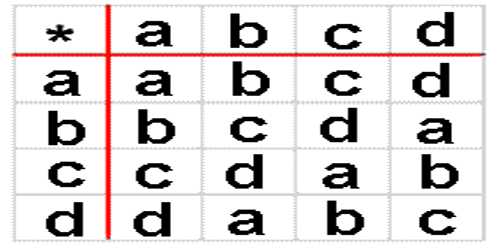Binary operations involve two quantities and include addition, subtraction, multiplication, division and exponentiation.

A binary operation f(x,y) is an operation that applies to two quantities or expressions x and y.

A binary operation on a nonempty set A is a map f:A×A->A such that

• f is defined for every pair of elements in A, and
• f uniquely associates each pair of elements in A to some element of A.

Examples of binary operation on A from A×A to A include addition (+), subtraction (-), multiplication (×) and division (÷).

Given a binary operation on a set there might be no identity element. There might be many. There might be left identities which are not right identities and vice-versa. We tend to be familiar with the situation in which there is a unique identity. Also note that an identity (left or right or both) for one operation does not have to be an identity for another operation. Think of addition and multiplication on the reals where the identities are 0 and 1 respectively. This can be quite dangerous for unusual binary operations on familiar sets.

Law of Binary OperationBack to Top

Below you could see relationship between commutative and associative binary operation.

An operation that needs two inputs.

A simple example is the addition operation “+”:

In “2 + 3 = 5”, the operation is “+”, which takes the two values “2” and “3” and gives the result “5”.

Subtraction, multiplication and division are also binary operations, and there are many more.

The two inputs are called “operands”.Commutative Law

Let * be a binary operation on the set S.

* is said to be commutative in S if a, b ε S, a * b = b * a

Associative Law

Let * be a binary operation on the set S.

* is said to be an associative in S if a, b ε S, a * (b * c) = (a * b) * c.

Explanation

An algebraic operation on a set AA with two operands in a given order, hence a function from A×A→AA×A→A. Such an operator may be written in conventional function or prefix form, as f(a,b)f(a,b), occasionally in postfix form, as abωabω or (a,b)ω(a,b)ω, but more commonly in infix form as a⋆ba⋆b where ⋆⋆ is the operator symbol. Many arithmetic, algebraic and logical functions are expressed as binary operations, such as addition, subtraction, multiplication and division of various classes of numbers; conjunction, disjunction and implication of propositions.

A binary operation is partial if it is not defined on all pairs (a,b)∈A×A(a,b)∈A×A (as for example division by zero is not defined). Properties of binary operations which occur in many contexts include

Commutativity: a⋆b=b⋆aa⋆b=b⋆a;

Associativity: a⋆(b⋆c)=(a⋆b)⋆ca⋆(b⋆c)=(a⋆b)⋆c;

Idempotence: a⋆a=aa⋆a=a.